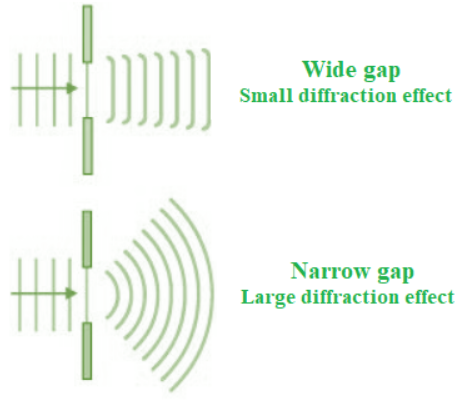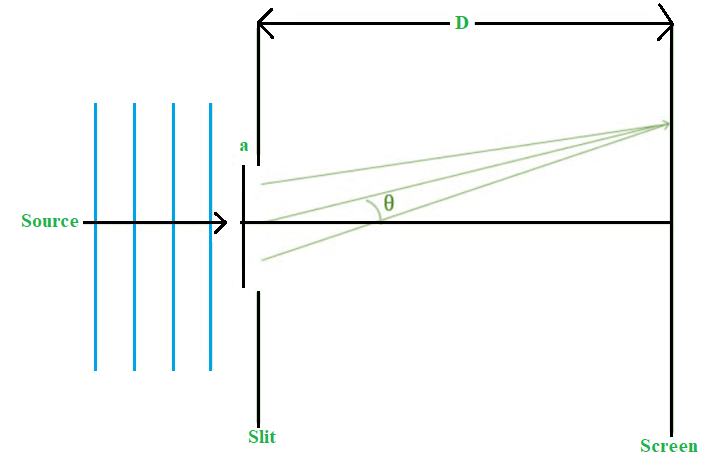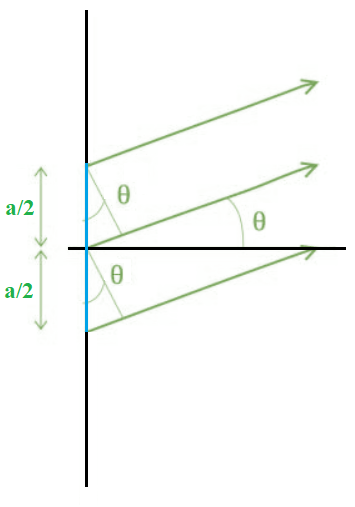Diffraction of light

• Last Updated : 03 Sep, 2021

Interference, refraction, reflection, and diffraction are all examples of processes that occur as light travels through the air. Diffraction of light occurs when it comes into contact with an obstruction. The wavefront on the opposite side of the aperture mimics the wave when light flows through a small opening that is equivalent in size to the wavelength of the light.

When light passes through a single slit with a width (w) on the order of the wavelength of the light, we can see single slit diffraction. The screen’s diffraction pattern will be at a distance L >> w from the slit. The intensity is proportional to the angle.

What is Diffraction?

The bending of light around corners such that it spreads out and illuminates regions, where a shadow is expected, is known as diffraction.

In general, it’s difficult to distinguish between diffraction and interference since they both happen at the same time. The diffraction of light is responsible for the silver line we see in the sky. A silver line appears in the sky as sunlight travels through or hits a cloud.

When Young’s double-slit experiment is substituted with a single narrow slit, a wide pattern with a brilliant area in the centre appears. There are alternating dark and bright areas on both sides of the centre. The intensity decreases as you move away from the centre. The single slit diffraction of light is discussed in depth in this article.

Single Slit Diffraction

We may see the bending phenomena of light, or diffraction, in the single-slit diffraction experiment, which causes light from a coherent source to interfere with itself and form a distinct pattern on the screen termed the diffraction pattern. When the sources are tiny enough to be comparable in size to the wavelength of light, diffraction occurs. This impact may be seen in the diagram below. The spreading out of big apertures is minor and almost imperceptible.Single Slit Diffraction

Formula for Single Slit Diffraction

We’ll denote the slit width a << D, and the distance between the slit and the screen is D.The angular location of any point on the screen will be determined by measuring from the slit centre, which splits the slit by a ⁄ 2 lengths. To explain the pattern, we’ll look at the state of black fringes first. Let us also split the slit into equal-width zones a ⁄ 2. Let’s take a look at a pair of rays that come from a ⁄ 2 distances apart, as illustrated below.The top two rays indicate the following route difference:

Δ L = (a ⁄ 2) sinθ

Note: Remember that this is only a viable computation if D is really large.

Any number of ray pairs that start at a distance of a ⁄ 2 from one another, such as the bottom two rays in the diagram, can be considered. Any arbitrary pair of rays separated by a ⁄ 2 can be taken into account. In a minute, we’ll discover how important this method is. The path difference must create destructive interference for a dark fringe; the path difference must be out of phase by λ ⁄ 2. (λ represents the wavelength)

For the first fringe,

Δ L = λ ⁄ 2 = a ⁄ 2 sinθ

λ = a sinθ

There is another beam at a distance of a ⁄ 2 that can create destructive interference for a ray coming from any point in the slit. As each ray originating from a point has a counterpart that produces destructive interference, there is destructive interference at θ = sin−1(λ ⁄ a). As a result, a dark fringe is created.

We may divide the slit into four equal portions of a ⁄ 4 and use the same rationale for the next fringe. As a result, for the second minima:

λ ⁄ 2 = a ⁄ 4 sinθ

2λ = a sinθ

Similarly, we may divide the slit into 2n parts for the nth fringe and utilise the following condition:

λ ⁄ 2 = a ⁄ 2n sinθ

n λ = a sinθ

The Central Maximum

The maxima are located between the minima, and the width of the central maximum is equal to the distance between the 1st order minima on both sides of the screen.

The position of the minima determined by y (as measured from the screen’s centre) is:

tanθ ≈ θ ≈ y ⁄ D

For small θ,

sinθ ≈ θ

⇒ λ = a sinθ ≈ a θ

Angular width,

θ = y/D = λa

Central maximum,

y = λ D a

The centre maximum’s width is just twice this amount.

Width of central maximum = 2λ D a

Angular width of central maximum = 2θ = 2λ a

Sample Questions

Question 1: What is the difference between phases?

The difference between any two waves or particles with the same frequency and originating from the same place is known as phase difference. It’s measured in radians or degrees.

Question 2: What conditions must be met for constructive interference to occur?

The path difference must be an integral multiple of the wavelength in order for constructive interference to occur.

Question 3: What are the requirements for harmful interference to occur?

The path difference must equal an odd integral multiple of half-wavelength for destructive interference to occur.

Question 4: What is temporal coherence, and what does it mean?